cancel
Showing results for
Did you mean:

Fabric is Generally Available. Browse Fabric Presentations. Work towards your Fabric certification with the Cloud Skills Challenge.Helper III

## Slicer for Units/Value and YTD/MAT

Hello,

I'd like to create a slicer based on my data and measures.
I'd like there to be a slicer for value aswell as for unit. Here is how my dashboard currently looks.I'd like the option to pick Units, and the same charts and calculations would be made.
Also, I would like to have the option to pick between YTD and MAT calculations. As seen from the screenshot, currently my dashboard is showing YTD, but I have made a MAT measure aswell, which I would like to enable using a slicer to switch between YTD and MAT.

Any idea how I can do this? Would appreciate any help. Thanks.

6 REPLIES 6Community Support

Hi  @Bobbys ,

I created some data: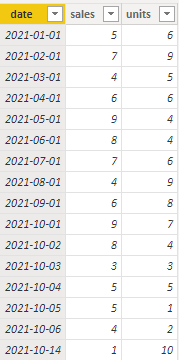Here are the steps you can follow：

1. Click Enter data to create a table Dynamic.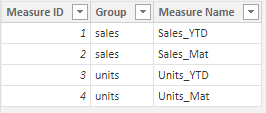2. Create measure.

``````Sales_YTD =
CALCULATE(SUM('amount_table'[sales]),FILTER(ALL('amount_table'),'amount_table'[date]>=DATE(YEAR(TODAY()),1,1)&&'amount_table'[date]<=TODAY()))``````
``Sales_Mat = CALCULATE(SUM('amount_table'[sales]),FILTER(ALL('amount_table'),'amount_table'[date]>=DATE(YEAR(TODAY()),MONTH(TODAY()),1)&&'amount_table'[date]<=TODAY()))``
``````Units_YTD =
CALCULATE(SUM('amount_table'[units]),FILTER(ALL('amount_table'),'amount_table'[date]>=DATE(YEAR(TODAY()),1,1)&&'amount_table'[date]<=TODAY()))``````
``Units_Mat = CALCULATE(SUM('amount_table'[units]),FILTER(ALL('amount_table'),'amount_table'[date]>=DATE(YEAR(TODAY()),MONTH(TODAY()),1)&&'amount_table'[date]<=TODAY()))``
``````Slice =
SWITCH(
SELECTEDVALUE('Dynamic'[Measure Name]),
"Sales_YTD",[Sales_YTD],
"Sales_Mat",[Sales_Mat],
"Units_YTD",[Units_YTD],
"Units_Mat",[Units_Mat])

``````

3. Result: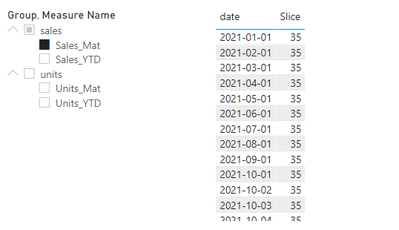Does this match your expected result.

Best Regards,

Liu Yang

If this post helps, then please consider Accept it as the solution to help the other members find it more quicklyHelper III

That's brilliant @v-yangliu-msft
Now, I might sound like a pain in the ass, but is there any way I can implement the previous year's YTD and MTD, when clicking on the slicer?

An example:So by clicking "Sales YTD" slicer, the table also updates with the YTD -1 numbers. And when I'd pick "Sales MAT", it would show Sales MAT and Sales MAT - 1. I have all these measures already calculated, so I have the numbers. I'd just like the table to update itself with the previous period of the measure picked in the slicer

Thanks again!!Community Support

Hi  @Bobbys ,

I created some data:Here are the steps you can follow：

1. Click Enter data to create a table Dynamic.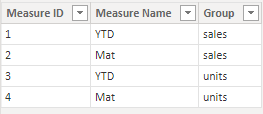2. Create measure.

``````Mat =
CALCULATE(SUM('Table'[amount]),FILTER(ALL('Table'),'Table'[date]>=DATE(YEAR(TODAY()),MONTH(TODAY()),1)&&'Table'[date]<=TODAY()&&'Table'[group]=MAX('Dynamic'[group])))``````
``````YTD =
CALCULATE(SUM('Table'[amount]),FILTER(ALL('Table'),'Table'[date]>=DATE(YEAR(TODAY()),1,1)&&'Table'[date]<=TODAY()&&'Table'[group]=MAX('Dynamic'[group])))``````
``````Measure_Slice =
SWITCH(
SELECTEDVALUE('Dynamic'[Measure Name]),
"YTD",[YTD],
"Mat",[Mat])``````
``````Flag =
IF(
MAX('Table'[group])=SELECTEDVALUE('Dynamic'[group]),1,0)``````

3. Place [group] and [Measure Name] of the Dynamic table into the slicer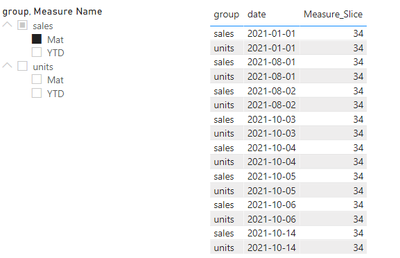4. Place [Flag] in the Filter of the table visual object, set is = 1, and apply filter.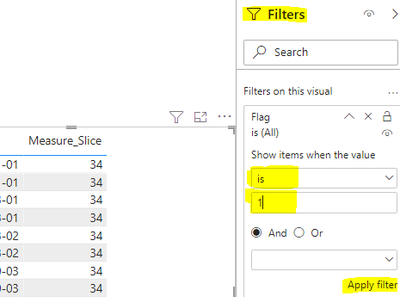5. Result:Best Regards,

Liu Yang

If this post helps, then please consider Accept it as the solution to help the other members find it more quicklyHelper III

Hello @v-yangliu-msft, thanks for the response.

In your table, the value for volume and sales value are in the same column, meanwhile I have one collumn for sales and one for volume. Therefore your DAX for YTD and MAT won't work on my data. This is how my table looks:Community Support

Hi  @Bobbys ,

I created some data:[Mat] and [YTD] are two measures.

Here are the steps you can follow：

1. Click Enter data to create a table Dynamic.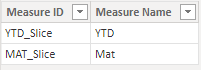2. Create measure.

``````Measure_Slice =
SWITCH(
MIN('Dynamic'[Measure ID]),
"YTD_Slice",[YTD],
"MAT_Slice",[Mat])``````

3. Use [Measure_Name] as the slicer

4. Result:

When the slicer selects Mat, the value of Measure[Mat] is displayed.When the slicer selects YTD, the value of Measure[YTD] is displayed.Best Regards,

Liu Yang

If this post helps, then please consider Accept it as the solution to help the other members find it more quicklyHelper III

Absolutely brilliant @v-yangliu-msft.
Is there any way I can have this for units aswell?
So it would look something like this:

* Sales
* YTD
* MAT

* Units
* YTD
* MAT

It would pretty much be that sales and units would be the slices, and then YTD or MAT would be the slicer inside the slicer again. Any way to get this?

Best regards and thanks a lotAnnouncements#### Power BI Monthly Update - November 2023

Check out the November 2023 Power BI update to learn about new features.#### Fabric Community News unified experience

Read the latest Fabric Community announcements, including updates on Power BI, Synapse, Data Factory and Data Activator.#### The largest Power BI and Fabric virtual conference

130+ sessions, 130+ speakers, Product managers, MVPs, and experts. All about Power BI and Fabric. Attend online or watch the recordings.Top Solution Authors
Top Kudoed Authors
Users online (2,361)